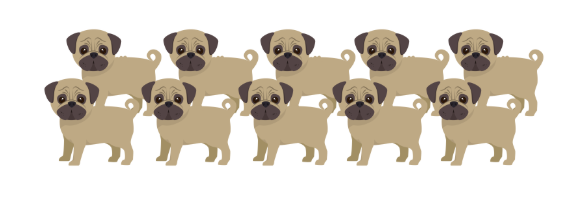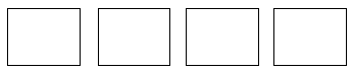# Multiplication and Division, Part 1

## Objective

Identify and create situations involving unknown group size and find group size in situations.

## Common Core Standards

### Core Standards

?

• 3.OA.A.1 — Interpret products of whole numbers, e.g., interpret 5 × 7 as the total number of objects in 5 groups of 7 objects each. For example, describe a context in which a total number of objects can be expressed as 5 × 7.

• 3.OA.A.2 — Interpret whole-number quotients of whole numbers, e.g., interpret 56 ÷ 8 as the number of objects in each share when 56 objects are partitioned equally into 8 shares, or as a number of shares when 56 objects are partitioned into equal shares of 8 objects each. For example, describe a context in which a number of shares or a number of groups can be expressed as 56 ÷ 8.

• 3.OA.A.3 — Use multiplication and division within 100 to solve word problems in situations involving equal groups, arrays, and measurement quantities, e.g., by using drawings and equations with a symbol for the unknown number to represent the problem.

## Criteria for Success

?

1. Represent the number of groups and size of groups in a picture.
2. Divide a number of objects into a given number of groups in order to find the size of each group.
3. Write a multiplication equation to represent a given situation or picture where the unknown is the size of each group, including the number of objects in each row of an array (MP.2).
4. Draw a picture to represent a given multiplication equation where the missing factor is the size of each group, including the number of objects in each row of an array (MP.2).

## Tips for Teachers

?

• The following material is needed for today's lesson: Counters

#### Remote Learning Guidance

If you need to adapt or shorten this lesson for remote learning, we suggest prioritizing Anchor Task 2 (benefits from worked example) and Anchor Task 3 (can be done independently). Find more guidance on adapting our math curriculum for remote learning here.

#### Fishtank Plus

• Problem Set
• Student Handout Editor
• Vocabulary Package

?

### Problem 1

Split 18 counters equally into two groups.

a. How many counters do you have in each group?

b. Write a multiplication equation to represent this situation.

### Problem 2

a.  The pet store owner has 30 dog treats and feeds the same amount of treats to each of the dogs below.i. Write a multiplication equation to represent this problem.

ii. How many treats does he feed to each dog?

b. Mr. Peddie is arranging 20 chairs into 4 rows in the gymnasium for the spring performance.

i. Write a multiplication equation to represent this problem.

ii. How many chairs does he put in each row?

### Problem 3

Draw a picture or write a story to represent this multiplication situation. Then find the solution.

$5\times$ ____ $=10$

## Problem Set & Homework

#### Discussion of Problem Set

• What was similar about #8 and #9? What was different?
• Why was the unknown factor always the second one in our multiplication equations today?
• How did your visual models in #11 and #12 help you to determine the unknown factor? Did anyone use a different strategy to solve?

?

### Problem 1

There are 20 glue sticks for the class. The teacher splits them into 4 equal groups. Draw the number of glue sticks in each group.#### References

EngageNY Mathematics Grade 3 Mathematics > Module 1 > Topic B > Lesson 4Exit Ticket, Question #1

Grade 3 Mathematics > Module 1 > Topic B > Lesson 4 of the New York State Common Core Mathematics Curriculum from EngageNY and Great Minds. © 2015 Great Minds. Licensed by EngageNY of the New York State Education Department under the CC BY-NC-SA 3.0 US license. Accessed Dec. 2, 2016, 5:15 p.m..

Modified by The Match Foundation, Inc.

### Problem 2

Which expression can be used to find the number of glue sticks in each group?

A.    $4 \times 20 =$ ____

B.    $20 – 4 =$ ____

C.    $4\times$ ____ $=20$

D.    $4+$ ____ $=20$

#### References

EngageNY Mathematics Grade 3 Mathematics > Module 1 > Topic B > Lesson 4Exit Ticket, Question #1

Grade 3 Mathematics > Module 1 > Topic B > Lesson 4 of the New York State Common Core Mathematics Curriculum from EngageNY and Great Minds. © 2015 Great Minds. Licensed by EngageNY of the New York State Education Department under the CC BY-NC-SA 3.0 US license. Accessed Dec. 2, 2016, 5:15 p.m..

Modified by The Match Foundation, Inc.

### Problem 3

Draw a picture to show $3\times$ ____ $=15$. Then, fill in the blank to make a true multiplication equation.

$3\times$ ____ $=15$

#### References

EngageNY Mathematics Grade 3 Mathematics > Module 1 > Topic B > Lesson 4Exit Ticket, Question #2

Grade 3 Mathematics > Module 1 > Topic B > Lesson 4 of the New York State Common Core Mathematics Curriculum from EngageNY and Great Minds. © 2015 Great Minds. Licensed by EngageNY of the New York State Education Department under the CC BY-NC-SA 3.0 US license. Accessed Dec. 2, 2016, 5:15 p.m..

Modified by The Match Foundation, Inc.

### Mastery Response

?